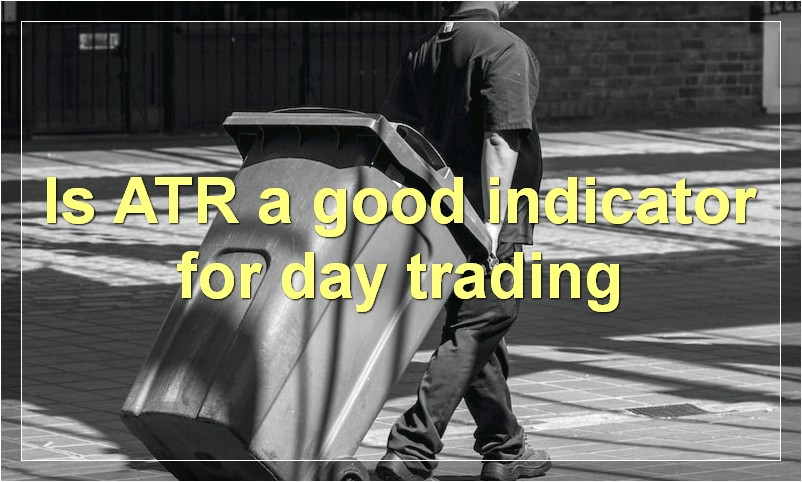# Average True Range: Everything You Need To Know

If you’re an active trader, then you know that volatility is key. And if you want to measure volatility, then you need to know about Average True Range.

## What is the average true range formulaThe average true range (ATR) is a technical indicator that measures market volatility. It was developed by J. Welles Wilder in his 1978 book, New Concepts in Technical Trading Systems.

The ATR is typically based on 14 periods and can be calculated on an intraday, daily, weekly, or monthly basis. The ATR does not provide an indication of price direction, but only measures the degree of price volatility. Wilder recommended a 14-period ATR to capture the majority of major price moves.

The ATR is calculated as the greatest of the following:

Current high less the current low
Current high less the previous close
(Absolute value of) the current low less the previous close

The first calculation is simply the difference between the highest and lowest prices of the current period. The second calculation takes into account the previous close. If it is greater than the current high-low range, then it is used instead. The third calculation is identical to the second except that it uses the absolute value of the difference between the current low and previous close, which eliminates any negative values that might skew the average.

Once these three values are determined, they are added together and divided by 14 to arrive at the ATR value.

## How do you calculate average true range

There are a few steps involved in calculating the average true range. First, you need to find the true range for each period. The true range is simply the highest high minus the lowest low, or the absolute value of the difference between the high and the low.

Next, you take the average of the true ranges over a specific number of periods. For example, if you’re using a 14-day ATR, you would add up the true ranges for the past 14 days and divide by 14. Doing this gives you the average true range for the past 14 days.

## What is the difference between ATR and other volatility indicators

ATR is the average true range, a measure of volatility. It is generally used by traders to determine whether a market is too volatile to enter or exit. Other volatility indicators include the standard deviation and Bollinger Bands.

## What are some common uses for ATR

ATR is commonly used as a measure of risk. It can give you an idea of how much you could lose on a trade, which can help you make better decisions about which trades to take. ATR can also be used to set stop-losses and take-profits.

## Is ATR a good indicator for day tradingATR, or Average True Range, is a technical indicator that measures the volatility of a security. It is often used by day traders to determine the best times to enter and exit trades.

ATR is calculated using a simple formula:

ATR = (High – Low) / 2

The ATR indicator is displayed as a single line on a price chart. The indicator can be used to help day traders set stop-loss orders and take-profit levels.

When the ATR is rising, it indicates that the security is becoming more volatile. This can be a good time to enter into trades. Conversely, when the ATR is falling, it indicates that the security is becoming less volatile. This can be a good time to exit trades.

The ATR indicator can be a useful tool for day traders. It can help them to determine the best times to enter and exit trades.

## What time frames are best for using ATR

There is no one definitive answer to this question as different traders will have different opinions based on their own experiences and preferences. However, some common time frames that are often used for ATR trading include the 4-hour, daily, and weekly charts. These time frames can provide enough information to help make informed trading decisions, while not being so overwhelming that it becomes difficult to interpret the data. Ultimately, it is up to each individual trader to determine which time frame works best for them.

## How can ATR be used to set stop losses

ATR can be used to set stop losses in a variety of ways. The most common way is to use the ATR as a percentage of the current price. For example, if the ATR is 50 pips and the current price is 1.2500, a stop loss could be placed at 1.2450. Another way to use the ATR is to set a stop loss at a certain number of ATRs below the current price. For example, if the ATR is 50 pips and the current price is 1.2500, a stop loss could be placed two ATRs below the current price, which would be 1.2000.